• fir数字滤波器matlab实现
2021-04-20 12:30:53

《FIR数字滤波器的Matlab实现[高等教育]》由会员分享，可在线阅读，更多相关《FIR数字滤波器的Matlab实现[高等教育](8页珍藏版)》请在人人文库网上搜索。

1、第7章 FIR数字滤波器的Matlab实现7.1 实验目的l 学习用窗函数法设计FIR数字滤波器的原理及其设计步骤；l 学习编写数字滤波器的设计程序的方法，并能进行正确编程；l 根据给定的滤波器指标，给出设计步骤。 7.2 实验原理及实例分析7.2.1 FIR低通数字滤波器的设计原理如果系统的冲激响应为已知，则系统的输入/输出关系为：对于低通滤波器，只要设计出低通滤波器的冲激响应函数，就可以由上式得到系统的输出了。假设所希望的数字滤波器的频率响应为，它是频域的周期函数，周期为2，那么它与相对应的傅立叶系数为以为冲激响应的数字滤波器将具有频域响。但是将作为滤波器脉冲响应有两个问题：(1) 它是无。

2、限长的，与FIP滤波器脉冲响应有限长这一前提不一致(2) 它是非因果的，对此，要采取以下的措施，(1) 将截短 (2)将其往右平移，由此得到的实际频域响应，与理想频域响应相近，但不完全一致。理论证明上述现象是对进行简单截短处理的必然结果，一般称为吉布斯现象，为尽可能的减少吉布斯现象，应对进行加窗截取，即以作为FIR滤波器的系数。常用的窗函数有矩形窗、海明窗和布莱克曼窗等。7.2.2 用窗函数法设计FIR滤波器Matlab设计FIR滤波器有多种方法和对应的函数，见表71。表71 matlab设计FIR滤波器的方法和函数方法描述函数窗方法使用窗函数和逆傅立叶变换实现fir1,fir2,kaiser。

3、ord等多带方法包含子带频率域firls，remez等最小二乘法使用最小二乘法将整个频率域上的错误几率压缩到最小fircls ，fircls1等任意响应法使用任意响应，包括非线性相位以及复滤波器cremez等余弦法使用三角函数的低通响应firrcos等窗函数方法不仅在数字滤波器的设计中占有重要的地位，同时可以用于功率谱的估计，从根本上讲，使用窗函数的目的就是消除由无限序列的截短而引起的Gibbs现象所带来的影响。窗函数设计线性相位FIR滤波器步骤如下：(1) 确定数字滤波器的性能要求，临界频率，滤波器单位脉冲响应长度N(2) 根据性能要求，合理选择单位脉冲响应h(n)的奇偶对称性，从而确定理想。

4、频率响应的幅频特性和相频特性(3) 求理想单位脉冲响应，在实际计算中，可对采样，并对其求IDFT的，用代替(4) 选择适当的窗函数w(n)，根据求所需设计的FIR滤波器单位脉冲响应(5) 求，分析其幅频特性，若不满足要求，可适当改变窗函数形式或长度N，重复上述设计过程，以得到满意的结果FIR1.m调用格式：a) b=fir1(N,wn)b) b=fir1(N,wn,hign)N为滤波器的阶次，wn是通带截止频率，其值在01之间，1对应抽样频率的一半；b是设计好的滤波器系数h(n)。对于格式a)，若wn是标量，则用来设计低通滤波器，若wn是1L的向量，则用来设计带通(带阻)滤波器。其格式为：b=。

5、fir1(N,wn,DC-1)或b=fir1(N,wn,DC-0),其中，前者保证第一个带为通带，后者保证第一个带为阻带。b)用来设计高通滤波器。用于产生窗函数的matlab文件有如下8个：bartlett.m(巴特利特窗)blackman.m(布莱克曼窗)rectangle.m(矩形窗)hamming.m(汉明窗)hanning.m(汉宁窗)triang.m(三角窗)chebwin.m(切比雪夫窗)Kaiser.m(凯泽窗)【实例7-1】 设计一个34阶的高通滤波器，截止频率为0.48，使用具有30dB波纹的chebyshev窗。解：源程序如下：b=fir1(34,0.48,high,che。

6、bwin(35,30);freqz(b,1,512)其响应波形如图7-1所示。图71 带通FIR滤波器【实例7-2】设计一个24阶FIR带通滤波器，通带频率。解：源程序如下：wn=0.35 0.65;N=24;b=fir1(2*N,wn,DC-0);% DC-0表示第一个带为阻带，这样设计出来的滤波器为带通滤波器。freqz(b,1,512)图72 带通FIR滤波器【实例7-3】 设计具有下面指标的低通FIR滤波器由于其最小阻带衰减为50dB，因此可以选择hamming窗来实现这个滤波器，因为它具有较小的过渡带。解：MATLAB源程序为%数字滤波器指标wp=0.2*pi;ws=0.3*pi;t。

7、r_width=ws-wp; %通带宽度N=ceil(6.6*pi/tr_width)+1; %滤波器长度if rem(N,2)=0N=N+1;endNw=N; %滤波器长度为奇数n=0:1:Nw-1;wc=(ws+wp)/2; %截止频率alpha=(Nw-1)/2;m=n-alpha+0.00001; %如果不加0.00001，当n= alpha 时，m为0，下式分母为0，不能执行hd=sin(wc*m)./(pi*m); %理想滤波器的冲激响应% 生成hamming窗w_ham=(hamming(Nw);% 频域图像的绘制h=hd.*w_ham; % 滤波器实际冲激响应freqz (h,。

8、1)figure(2);subplot(2,2,1),stem(n,hd);title(理想脉冲响应)axis(0 Nw-1 -0.3 0.3);xlabel(n);ylabel(hd(n)subplot(2,2,2),stem(n,w_ham);title(hamming窗)axis(0 Nw-1 -0.3 1.2);xlabel(n);ylabel(w(n)subplot(2,2,3),stem(n,h);title(实际脉冲响应)axis(0 Nw-1 -0.3 0.3);xlabel(n);ylabel(h(n)其响应波形如图7-2所示。图73 hamming窗函数设计FIR滤波器【实。

9、例7-4】设带通滤波器的指标为选择Blackman窗来实现这个滤波器。解：MATLAB源程序为% 数字滤波器指标ws1=0.2*pi; wp1=0.35*pi;ws2=0.65*pi; wp2=0.8*pi;As=60;tr_width=min(wp1-ws1),(wp2-ws2); % 过渡带带宽N=ceil(11*pi/tr_width)+1;if rem(N,2)=0N=N+1;endNw=N;n=0:1:Nw-1;wc1=(ws1+wp1)/2;wc2=(ws2+wp2)/2;alpha=(Nw-1)/2;m=n-alpha+0.00001; %如果不加0.00001，当n= alph。

10、a 时，m为0，下式分母为0，不能执行hd=sin(wc2*m)./(pi*m)- sin(wc1*m)./(pi*m); %理想滤波器的冲激响应% 生成blackman窗w_bla=(blackman(M);h=hd.*w_bla;% 频域图像的绘制freqz(h,1)figure(2);subplot(2,2,1),stem(n,hd);title(idael impulse response)axis(0 Nw-1 -0.4 0.5);xlabel(n);ylabel(hd(n)subplot(2,2,2),stem(n,w_bla);title(blackman window)axis。

11、(0 Nw-1 0 1.1);xlabel(n);ylabel(w(n)subplot(2,2,3),stem(n,h);title(actual impulse response)axis(0 Nw-1 -0.4 0.5);xlabel(n);ylabel(h(n)其响应波形如图7-4所示。图74 blackman窗函数设计FIR滤波器7.3 编程练习1. 设计一个48阶FIR带阻滤波器，通带频率。2.用窗函数设计一个线性相位FIR高通滤波器，并满足性能指标：通带边界频率,阻带边界频率，阻带衰减不小于40db。提示：参考例73，4；首先根据阻带衰减，选择合适的窗函数，见课本P342，表7-3。再根据P345，式7-74写出理想滤波器的冲激响应hd 。8辅导工具a。

更多相关内容
• FIR滤波器的相关知识以及生成所需要用到的matlab函数，具体生成例程。
• 本程序是窗函数法设计FIR数字滤波器Matlab程序——海明窗
• 基于Matlab的二维FIR数字滤波器的设计.pdf
• 基于MATLABFIR数字滤波器仿真设计研究 基于MATLABFIR数字滤波器仿真设计研究 基于MATLABFIR数字滤波器仿真设计研究 基于MATLABFIR数字滤波器仿真设计研究 基于MATLABFIR数字滤波器仿真设计研究 基于MATLAB...
• 文中针对传统FIR滤波器设计方法繁琐，设计步骤和过程繁杂，且设计好滤波器之后，不能直观快速修改滤波器参数来观察滤波器变化，设计了基于MATLABFIR数字滤波器MATLAB功能强大，在进行有限脉冲响应滤波器（FIR）...
• 本程序是窗函数法设计FIR数字滤波器Matlab程序——矩形窗
• matlab实现FIR数字滤波器，内附matlab源码matlab
• 提出一种改进粒子群优化算法(IMPSO)的FIR滤波器设计方法。该方法计算量小，整定时间短，并能有效克服早熟收敛的问题。
• 数字滤波器的工作原理为，通过一个离散数字系统，对输入的数字信号进行运算，处理，变换等各种数字信号操作，从而实现改变数字输入序列的频谱特性或者时域波形，将有用的信号频谱分量进行保留，消除无用的信号频谱...

目录

一、理论基础

二、案例背景

三、MATLAB核心代码

四、仿真结论分析

# 一、理论基础

根据快速傅里叶变换的数学原理可知，对于一个任意长度的序列，通过对其频谱特性进行等间隔的数字抽烟操作，然后通过离散傅里叶反变换，便可获得一个有限长的数字序列。这个 有限长序列的周期性是原序列的主值序列，而且频率特性和原序列的频率特性相似。因此，对于一个理想的频率响应函数Hd(e)，其响应的单位抽样响应函数为h(n)，对频率响应函数Hd(e)所在的单位圆上做等间隔划分，即等间隔频率抽样，从而得到频率抽样值H(k)，最后对H(k)进行离散傅里叶反变换，即：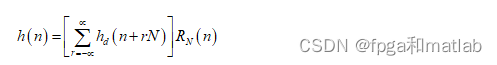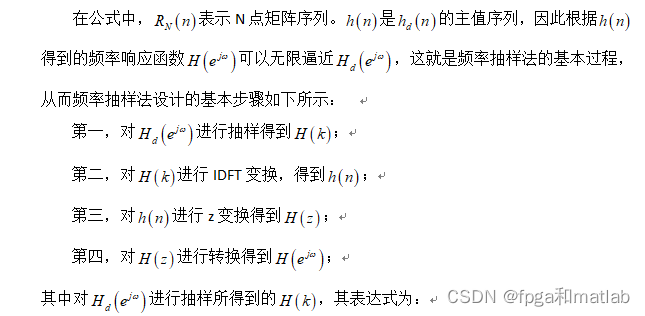# 二、案例背景

滤波器的含义，就是指对输入信号有着消除噪声和平滑功能的系统。根据处理信号种类的不同，将处理连续信号的滤波器称为模拟滤波器，将处理离散信号的滤波器称为数字滤波器。随着通信多媒体技术的飞速发展，数字滤波器成为数字信号处理领域中一个越来越重要的组成部分，其广泛应用在信息通信、语音传输、自适应控制等众多领域。从结构上分，数字滤波器可以分为FIR数字滤波器和IIR数字滤波器两种基本类型。其中，FIR滤波器的设计方法有许多种，如窗函数设计法、频率取样法和等波纹最佳逼近法，本文主要针对FIR滤波器的实现原理和设计方法进行了研究。

数字滤波器本质上讲是一种通过有限精度算法来运算的线性时不变离散系统，其具有特定的传输选择性，数字滤波器的输入和输出信号均为离散数字信号。数字滤波器的工作原理为，通过一个离散数字系统，对输入的数字信号进行运算，处理，变换等各种数字信号操作，从而实现改变数字输入序列的频谱特性或者时域波形，将有用的信号频谱分量进行保留，消除无用的信号频谱分量。数字滤波器，在功能上可以分为低通数字滤波器、高通数字滤波器、带通数字滤波器以及带阻数字滤波器四种类型。相对于模拟滤波器，数字滤波器具有高精度，高稳定性，高灵活性等优势。

# 三、MATLAB核心代码

clc;
clear;
close all;

N = 63;
NN= 31;
H =[ones(1,(N-NN)/2),zeros(1,NN),ones(1,(N-NN)/2)];

k = 0:(N-1)/2;k1=(N+1)/2:(N-1);
A =[exp(-j*pi*k*(N-1)/N),exp(j*pi*(N-k1)*(N-1)/N)];
HK= H.*A;
hn= ifft(HK);

freqz(hn,1,512);
figure;
stem(real(hn),'.');

xlabel('n');
ylabel('h(n)'); 
clc;
clear;
close all;

N = 63;
NN= 51;
H =[ones(1,(N-NN)/2),zeros(1,NN),ones(1,(N-NN)/2)];
k = 0:(N-1)/2;k1=(N+1)/2:(N-1);
A =[exp(-j*pi*k*(N-1)/N),exp(j*pi*(N-k1)*(N-1)/N)];
HK= H.*A;
hn= ifft(HK);

freqz(hn,1,512);
figure;
stem(real(hn),'.');
line([0 35],[0 0]);
xlabel('n');
ylabel('h(n)');

Ts=1/1000  ;%设置采样频率

t=0:Ts:3*pi;
y=sin(2*pi*t)+cos(400*pi*t);
figure;
plotspec(y,Ts);

y_Win=filter(hn,1,y);
figure;
plotspec(y_Win,Ts);



# 四、仿真结论分析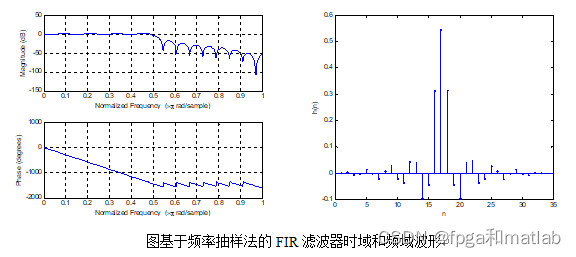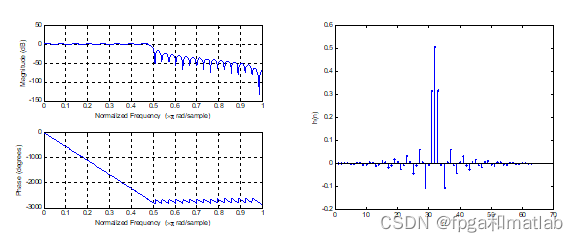从图仿真结果可知，频率抽样法所设计的FIR滤波器，其滤波性能是随着滤波器阶数的增加而增加的，因此，当阶数较大的时候，其硬件实现难度较大。

A25-25

展开全文matlab FIR滤波器
• 基于MATLAB GUI的FIR数字滤波器设计与仿真.pdf
• 2．4 FIR数字滤波器MATLAB实现 6 2．5不同阶数N的窗函数FIR数字滤波器 11 第三章 FPGA简介 14 第四章 基于FPGA的FIR滤波器设计 17 4．1加法器设计 17 4．2乘法器设计 17 4．3 FIR滤波器设计 18FIR数字滤波器
• 如模拟滤波器对连续时间信号的这种运算，在数学上是用线性常系数微分方程确定的。用拉普拉斯变换可在S域内进行这些运算的分析。用傅立叶变换则在f域内分析。同样的，可用常系数线性方程来定义离散时间信号的线性变.....

1 背景

在线性系统中，信号滤波过程一般定义为，当输入波形通过一个系统时，对它作一个线性运算，在时间域上这种变换如像内插，外插微分和积分，在频率域上这种变换则如低通滤波或平滑，带通滤波，谱设计和谱分析。如模拟滤波器对连续时间信号的这种运算，在数学上是用线性常系数微分方程确定的。用拉普拉斯变换可在S域内进行这些运算的分析。用傅立叶变换则在f域内分析。同样的，可用常系数线性方程来定义离散时间信号的线性变换，并且用二变换来分析和合成离散时间滤波器。

## 1.1 数字滤波器的研究背景和意义

当今，数字信号处理技术正飞速发展，它不但自成一门学科，更是以不同形式影响和渗透到其他学科；它与国民经济息息相关，与国防建设紧密相连；它影响或改变着我们的生产、生活方式，因此受到人们普遍的关注。

数字化、智能化和网络化是当代信息技术发展的大趋势，而数字化是智能化和网络化的基础，实际生活中遇到的信号多种多样，例如广播信号、电视信号、雷达信号、通信信号、导航信号等等。上述这些信号大部分是模拟信号，也有小部分是数字信号。模拟信号是自变量的连续函数，自变量可以是一维的，也可以是二维或多维的。大多数情况下一维模拟信号的自变量是时间，经过时间上的离散化（采样）和幅度上的离散化（量化），这类模拟信号便成为一维数字信号。因此，数字信号实际上是数字序列表示的信号，语音信号经采样和量化后，得到的数字信号是一个一维离散时间序列；而图像信号的经采样和量化后，得到的是数字信号是一个二维离散空间序列。

数字滤波技术是数字信号分析、处理技术的重要分支。无论是信号的获取、传输，还是信号的处理和交换都离不开滤波技术，它对信号安全可靠和有效灵活地传输时至关重要的。在所有的电子系统中，使用最多技术最复杂的要算数字滤波器了。数字滤波器的优劣直接决定产品的优劣。

## 1.2 数字滤波器的发展及其现状

数字滤波是从20世纪60年代发展起来的，当时主要采用计算机模拟的方法研究数字滤波问题。到了70年代，开始将微处理器应用于数字滤波。但是由于微处理器速度不高，在很多场合都难以实现实时处理。随着VLSI技术的发展，使用硬件来实现数字滤波器已成为可能。80年代，通用数字信号处理器的成熟和大量涌现，使得音频范围内数字滤波技术迅速得到广泛的应用。近些年来，除了不断提高通用信号处理器的速度和功能外，还出现了许多工作频率达100MHZ以上的专用芯片和积木式部件，从而使数字滤波技术进入视频实时处理阶段。

数字滤波在通信、图像编码、语言编码、雷达等许多领域中有着十分广泛的应用。目前，数字信号滤波器的设计图像处理、数据压缩等方面的应用取得了令人瞩目的进展和成就。近年来迅速发展起来的小波理论，由于其局部分析性能的优异在图像处理中的应用研究，尤其是在图像压缩、图像去噪等方面的应用研究。

## 3 FIR数字滤波器的设计与实现

FIR数字滤波器以其良好的线性特性被广泛应用于现代电子通信系统中，是数字信号处理的重要内容之一。Matlab软件含有多种现成的函数和工具箱,在滤波器设计时只需要调用这些窗函数修改关键数据即可实现不同要求的滤波器设计 。本文主要介绍的是采用窗函数法设计实现FIR带通滤波器。

## 3.1几种常用的窗函数

由上面的分析可知，窗函数法的设计关键是窗函数的选择。实际应用中比较常用的窗函数有：矩形窗函数、三角形((Bartlett)窗函数、汉宁(Hanning)窗函数、哈明(Hamming)窗函数、布莱克曼(Blackman)窗函数和凯塞(Kaiser)窗函数。下面首先介绍这些窗函数的特点。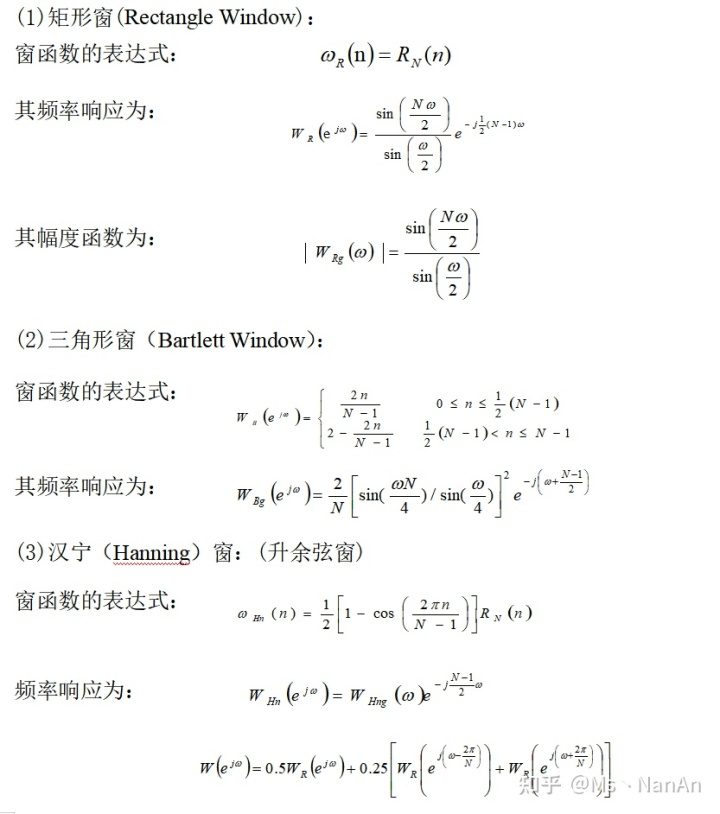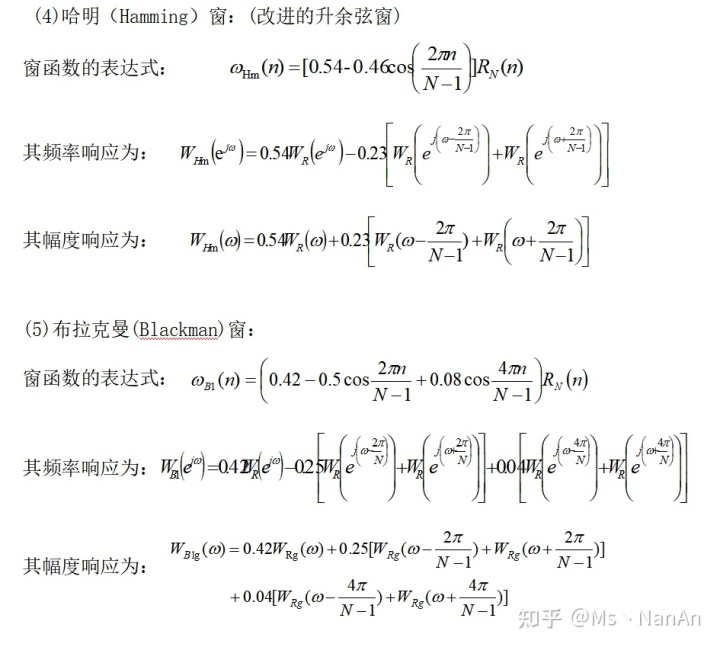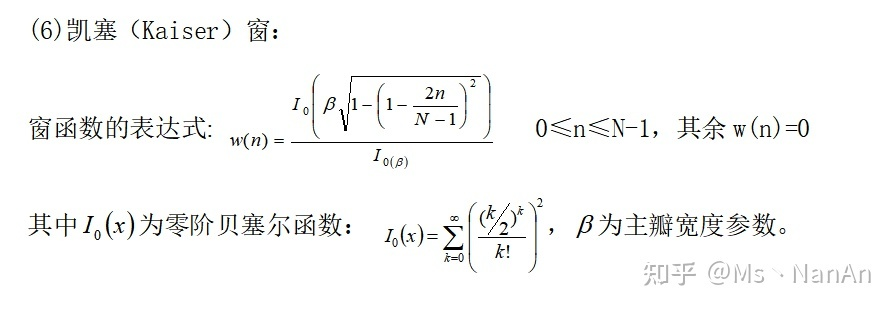这几种窗函数的参数比较如表1所示。

表1　六种窗函数的基本参数

 窗函数 旁瓣峰值幅度/dB 过渡带宽 阻带最小衰减/dB 矩形窗 -13 4π/N -12 三角形窗 -25 8π/N -25 汉宁窗 -31 8π/N -44 哈明窗 -41 8π/N -53 布莱克曼窗 -57 12π/N -74 凯塞窗 -57 10π/N -80

窗函数的选择原则是：

（1）具有较低的旁瓣幅度，尤其是第一旁瓣幅度；

（2）旁瓣幅度下降速度要大，以利增加阻带衰减；

（3）主瓣的宽度要窄，以获得较陡的过渡带。

通常上述三点很难同时满足。当选用主瓣宽度较窄时，虽然得到较陡的过渡带，但通带和阻带的波动明显增加：当选用最小的旁瓣幅度时，虽能得到匀滑的幅度响应和较小的阻带波动，但过渡带加宽。因此，实际选用的窗函数往往是它们的折衷。在保证主瓣宽度达到一定要求的条件下，适当牺牲主瓣宽度来换取旁瓣波动的减少。

## 3.2 利用窗函数设计FIR带通滤波器的设计步骤

本文以带通滤波器的设计为例，介绍窗函数法的实现。用窗函数法设计FIR数字滤波器的步骤如下：

1 根据对阻带衰减及过渡带的指标要求，选择窗函数的类型，并估计窗口长度N。先按照阻带衰减选择窗函数类型。原则是保证阻带衰减满足要求的情况下，尽量选择主瓣窄的窗函数。然后根据过渡带宽度估计窗口长度N。待求滤波器的过渡带宽度近似等于窗函数主瓣宽度，切近似与窗口长度N成反比，N≈，A取决于窗口类型，例如，矩形窗A=4，哈明窗的A=8等。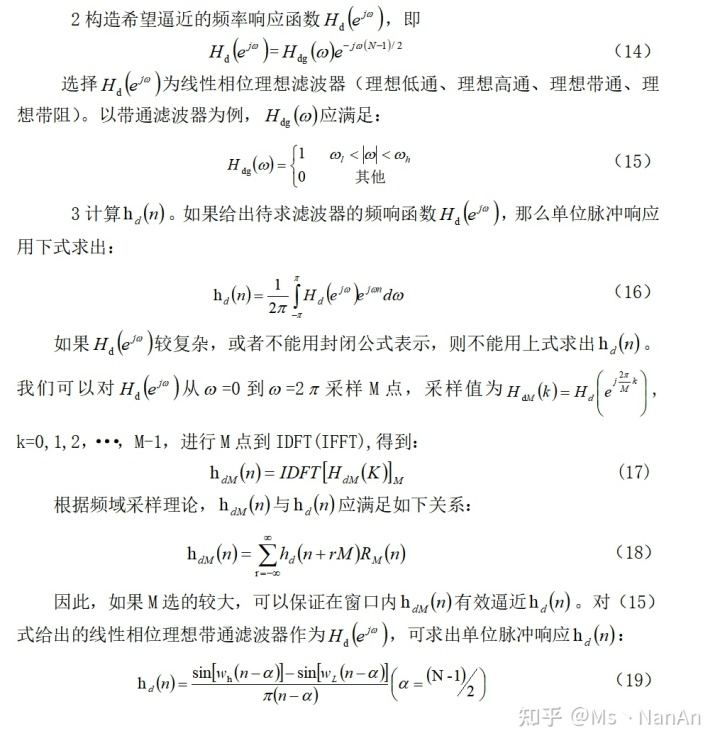## 3.3 基于MATLAB的FIR数字带通滤波器的仿真实现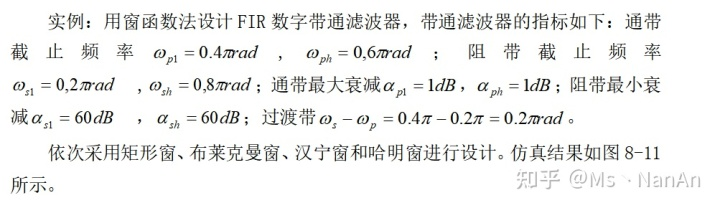实现程序如下：
clear all;close all;clc;
wpl=0.4*pi;wph=0.6*pi;wsl=0.2*pi;wsh=0.8*pi;
wd1=(wpl+wsl)/2;wd2=(wph+wsh)/2;
Bt=min(abs(wsl-wpl),abs(wsh-wph));
N=ceil(11*pi/Bt);
wn=[wd1/pi,wd2/pi];
h=fir1(N-1,wn,'bandpass', boxcar (N)); *// h=fir1(N-1,wn,'bandpass',blackman(N));//
*// h=fir1(N-1,wn,'bandpass',hanning(N));//
*// h=fir1(N-1,wn,'bandpass',hamming(N));//
wd=(wd2-wd1)/2;w0=0.5*pi;
M=(N-1)/2;nn=-M:M;n=nn+eps;
hd=2*sin(wd*n).*cos(w0*n)./(pi*n);           %理想冲激响应
w= boxcar (N)';                   *//w= blackman (N)';//
*//W= hanning(N)';//
*//w= hamming (N)';//
H=20*log10(abs(fft(h,1024)));   % 实际滤波器的分贝幅度特性
HH=[H(513:1024) H(1:512)];
subplot(221),stem(nn,hd,'k');
xlabel('n');title('(a)理想冲激响应');axis([-30 30 -0.4 0.5]);
subplot(222),stem(nn,w,'k');axis([-30 30 -0.1 1.2]);
title('(b)矩形窗');xlabel('n');           *// title('(b)布莱克曼窗');xlabel('n');//
*// title('(b)汉宁窗');xlabel('n');//
*// title('(b)哈明窗');xlabel('n');//
subplot(223),stem(nn,h,'k');
axis([-30 30 -0.4 0.5]);xlabel('n');title('(c)实际冲激响应');
w=(-512:511)/511;
subplot(224),plot(w,HH,'k');
axis([-1.2 1.2 -140 20]);xlabel('\omega/\pi');title('(d)滤波器分贝幅度特性');
set(gcf,'color','w');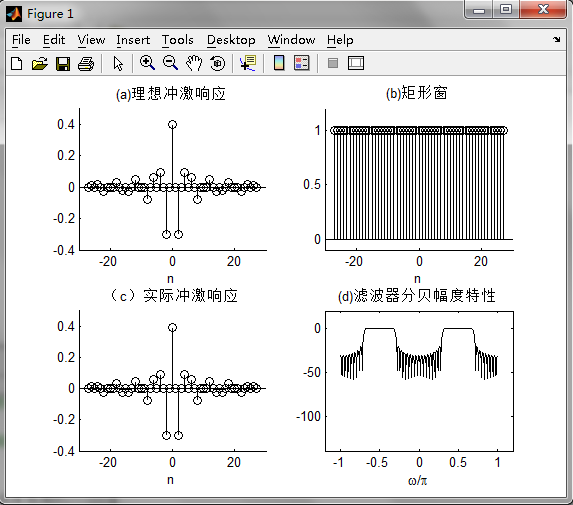图8为选用矩形窗窗设计带通滤波器的仿真结果图。图8（a）表示所要设计的滤波器的冲激响应图，图8（b）为所选用的窗函数——矩形窗。图8（c）为加窗后的实际冲击响应。图8（d）为设计得到的带通滤波器的幅度损耗函数图。由图可以看出，所设计的滤波器具有良好的带通滤波特性，满足设计任务要求。

矩形窗属于时间变量的零次幂窗。矩形窗使用最多，习惯上不加窗就是使信号通过了矩形窗。这种窗的优点是主瓣比较集中，缺点是旁瓣较高，并有负旁瓣，导致变换中带进了高频干扰和泄漏，甚至出现负谱现象。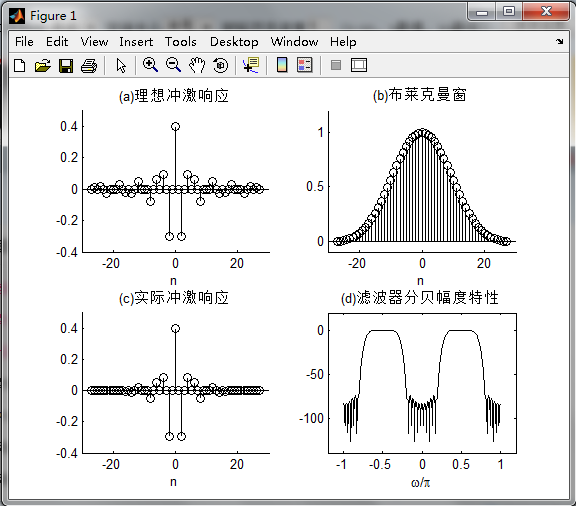图9为选用布莱克曼窗设计带通滤波器的仿真结果图。图9（a）表示所要设计的滤波器的冲激响应图，图9（b）为所选用的窗函数——布莱克曼窗。图9（c）为加窗后的实际冲击响应。图9（d）为设计得到的带通滤波器的幅度损耗函数图。由图可以看出，所设计的滤波器具有良好的带通滤波特性，满足设计任务要求。

布莱克曼窗增加一个二次谐波余弦分量，可进一步降低旁瓣，但主瓣宽度进一步增加，增加N可减少过渡带。布莱克曼窗设计的阻带最小衰减最大，达-74dB，但过渡带最宽，约为矩形窗的三倍。

图10为选用汉宁窗设计带通滤波器的仿真结果图。图10（a）表示所要设计的滤波器的冲激响应图，图10（b）为所选用的窗函数——汉宁窗。图10（c）为加窗后的实际冲击响应。图10（d）为设计得到的带通滤波器的幅度损耗函数图。由图可以看出，所设计的滤波器具有良好的带通滤波特性，满足设计任务要求。

汉宁窗主瓣加宽并降低，旁瓣则显著减小，从减小泄漏观点出发，汉宁窗优于矩形窗．但汉宁窗主瓣加宽，相当于分析带宽加宽，频率分辨力下降。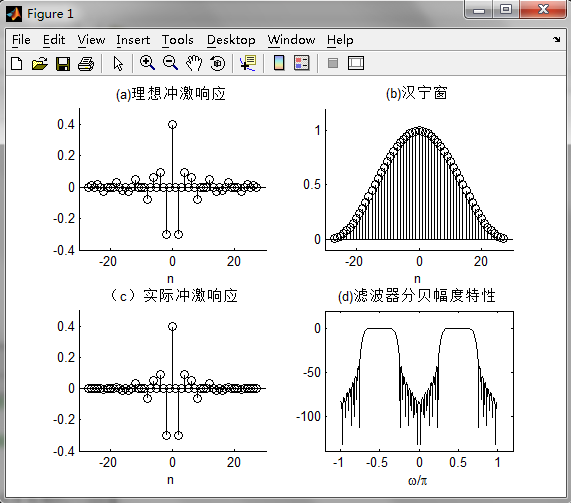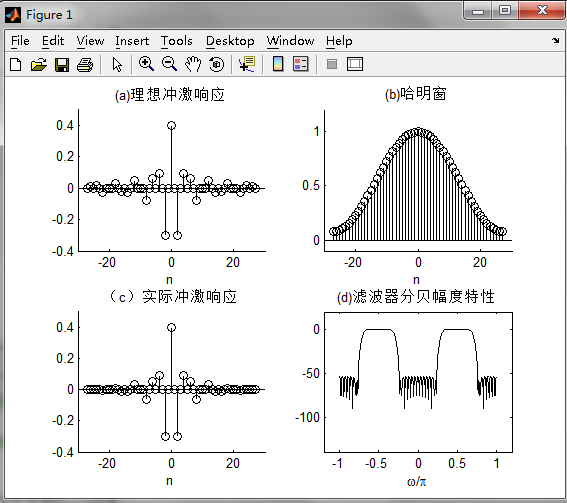图11为选用哈明窗设计带通滤波器的仿真结果图。图11（a）表示所要设计的滤波器的冲激响应图，图11（b）为所选用的窗函数——哈明窗。图9（c）为加窗后的实际冲击响应。图11（d）为设计得到的带通滤波器的幅度损耗函数图。由图可以看出，所设计的滤波器具有良好的带通滤波特性，满足设计任务要求。

哈明窗是对汉宁窗的改进，只是加权系数不同。哈明窗加权的系数能使旁瓣达到更小。在主瓣宽度（对应第一零点的宽度）相同的情况下，旁瓣进一步减小，可使99.96%的能量集中在主瓣内。

## 结论

本文首先介绍了数字滤波器的研究背景及其发展现状，然后介绍了FIR数字滤波器的设计原理。在理解设计方法的基础上，最后基于MATLAB软件利用窗函数法实现了FIR数字带通滤波器的设计。仿真结果表明，所设计的滤波器具有良好的滤波器特性，所设计的指标符合设计任务要求。

FIR数字滤波器的设计要取得好的逼近效果，除阶数N外，选择适合的窗函数也是重要方面的。通过对窗函数合理的选择，使得滤波器的通带衰减较小，阻带衰减较大；有较陡的过渡带，有利于对信号频带的选择。

在FIR滤波器中突出的性能中，也存在着一些不足：

1. 窗函数设计法是从时域出发的一种设计法，但一般技术指标是在频率给出的，其边界频率不容易控制，为达到较好的效果，需要多次调试。

2. 由于FIR滤波器的传输函数的极点固定在原点，所以只能采用较高的阶数来达到高的选择性。因此，它的经济性就相对差一些。

MATLAB由于本身带有多种工具箱和窗函数对于滤波器的设计有着多种设计方式，只需修改关键程序即可实现多种滤波器的设计。在图像处理、通讯、控制系统设计、测试和测量、财务建模和分析以及计算生物学等众多应用领域有着不可替代应用前进。

展开全文python 机器学习 java 人工智能
• 基于Matlab和FPGA的FIR数字滤波器设计及实现.pdf
• 数字信号处理实验FIR数字滤波器设计及软件实现.docxmatlab
• 基于MATLAB的IIR和FIR滤波器设计-实验4 FIR数字滤波器设计.doc IIR：在MATLAB中，可以用下列函数辅助设计IIR数字滤波器：1)利用buttord和cheb1ord可以确定低通原型巴特沃斯和切比雪夫滤波器的阶数和截止频率；2)...matlab
• matlab代码，直接运行出结果。FIR带通滤波器，并对数字信号进行带通滤波。建立含有3种频率和白噪声的信号。然后利用窗函数法设计
• Matlab辅助DSP实现FIR数字滤波器-Matlab辅助DSP实现FIR数字滤波器.rar 学习DSP一个阶段了，有收获但是也走了不少弯路，当初是找到论坛也是有学习DSP的原因，现在感觉这里真的不错，很有发展潜力，能在这里学到的...matlab
• FIR数字滤波器的最优化设计及MATLAB实现.pdf
• 本书以Altera公司的FPGA器件为开发平台，采用MATLAB及Verilog HDL语言为开发工具，详细阐述数字滤波器的FPGA实现原理、结构、方法以及仿真测试过程，并通过大量工程实例分析FPGA实现过程中的具体技术细节。...
• 本程序是窗函数法设计FIR数字滤波器Matlab程序——布莱克曼窗
• 1.实验目的 （1）掌握用窗函数法设计 FIR 数字滤波器的原理和方法。 （2）熟悉线性相位 FIR 数字滤波器特性。 （3）了解各种窗函数对滤波特性的影响。
• 湖北理工学院 毕业设计论文开题报告 1课题来源 实现数字滤波器的方法一般有两种一种方法是设计专用的数字硬件专用 的数字信号处理器或采用通用的数字信号处理器来实现另一种方法是把滤波器 所要完成的运算编成程序并...
• 山东大学DSP数字信号处理实验二十一 频率采样法设计FIR 数字滤波器 一、实验目的 1. 掌握用频率采样法设计线性相位 FIR 数字滤波器的方法。 2. 熟悉频率取样理论，熟悉内插函数及其应用。 3. 了解 FIR 数字滤波器的...

一、实验目的
1.  掌握用频率采样法设计线性相位 FIR  数字滤波器的方法。
2.  熟悉频率取样理论，熟悉内插函数及其应用。
3.  了解 FIR  数字滤波器的频率特性和相位特性，观察过渡带取样点对滤波器幅频特性的影响。
4．如何由线性相位条件决定采样值。

二、实验原理
1.前面介绍的窗函数法设计 FIR 滤波器是一种从时域出发的方法，而频率采样法则是一种从频域出发，对所给定的理想频率响应 H  (e ʲw ) 进行等间隔采样以后，用有限的采样点来设计所期望的滤波器（在频域内采用插值的办法逼近）。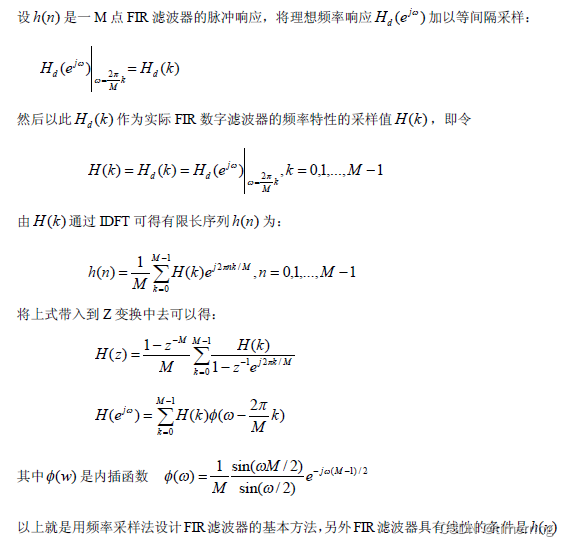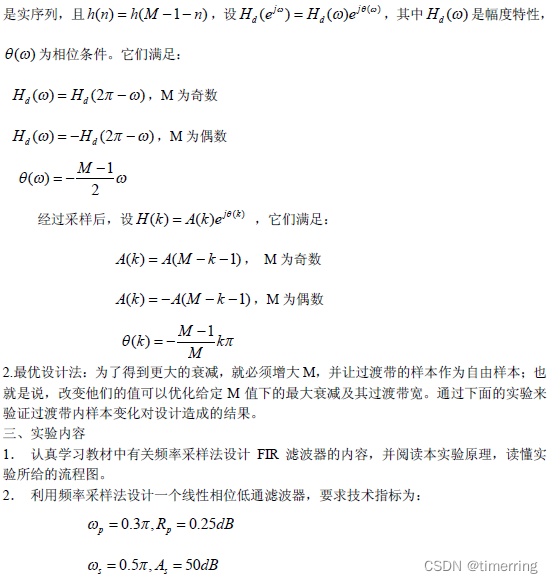取采样点为 M=20,画出所得滤波器的幅频响应，并检验阻带的最小衰减，编写 MATLAB 程
序实现。
提示：在进行频率采样时用到的 MATLAB 语句
A=[ones(1,M1+1),zeros(1,M2),ones(1,M1)];
这种通带和阻带之间没有过渡值的方法称为朴素设计法。
3．将采样点改为 M=60，重复实验内容 2，比较两者的差异。
参考流程图：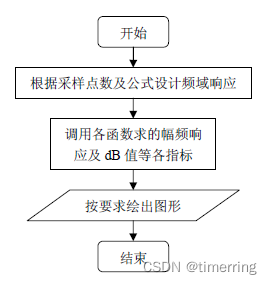4． 采用在通带和阻带之间插过渡点的方法，重复实验内容 2，3。并比较在进行插值后，
滤波器的性能的改进。并改变过渡点的值观察变化。
提示：插值的 MATLAB 语句  A=[ones(1,M1+1),T1,zeros(1,M2-2),T2,ones(1,M1)];
参考流程同上，只是在根据采样点数设计频率响应时应在过渡区间内加上一定数量的过渡点值，并分别加入不同数量或不同的数值，比较设计结果并分析。
四、思考题
①用频率采样法在选定频率样本的幅度和相位特性时应遵循什么条件？
②在频率采样法设计 FIR 滤波器时，为提高阻带衰减采取了什么方法？
③自己编程实验在过渡带内插入多个过渡点，重复实验内容，比较设计结果有何变化。

五、实验报告要求
1.简述实验目的及原理。
2.整理好经过运行并证明是正确的实验程序并加上注释。
3.试述频率采样法的优缺点。
4.简要回答思考题。

M=20;alpha=(M-1)/2;l=0:M-1;wl=(2*pi/M)*l; Hrs=[1,1,1,zeros(1,15),1,1];
Hdr=[1,1,0,0];wdl=[0,0.25,0.25,1];
k1=0:floor((M-1)/2);k2=floor((M-1)/2)+1:M-1;
angH=[-alpha*(2*pi)/M*k1,alpha*(2*pi)/M*(M-k2)];
H=Hrs.*exp(j*angH);h=real(ifft(H,M));
[db,mag,pha,grd,w]=freqz_m(h,1);
[Hr,ww,a,L]=Hr_Type2(h); subplot(221);
plot(wl(1:11)/pi,Hrs(1:11),'o',wdl,Hdr);
axis([0,1,-0.1,1.1]);title('Frequency Samples:M=20')
xlabel('frequency in pi units');ylabel('Hr(k)') subplot(222);stem(l,h);axis([-1,M,-0.1,0.3])
title('impulse response');xlabel('n');ylabel('h(n)');
subplot(223);plot(ww/pi,Hr,wl(1:11)/pi,Hrs(1:11),'o');
axis([0,1,-0.2,1.2]);title('amplitude response') xlabel('frequency in pi units');ylabel('Hr(w)')
subplot(224);plot(w/pi,db);axis([0,1,-60,10]);grid
title('magnitude response');xlabel('frequency in pi units');ylabel('Decibels');
子函数：
function [Hr,w,b,L] = Hr_Type2(h);
% Computes Amplitude response of Type-2 LP FIR filter
%
% [Hr,w,b,L] = Hr_Type2(h)
% Hr = Amplitude Response
%  w = frequencies between [0 pi] over which Hr is computed
%  b = Type-2 LP filter coefficients
%  L = Order of Hr
%  h = Type-2 LP impulse response
%
M = length(h);
L = M/2;
b = 2*[h(L:-1:1)];
n = [1:1:L]; n = n-0.5; w = [0:1:500]'*pi/500;
Hr = cos(w*n)*b';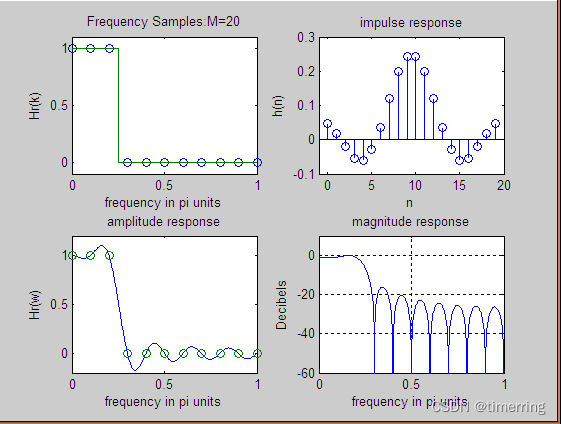M=60;alpha=(M-1)/2;l=0:M-1;wl=(2*pi/M)*l;
Hrs=[1,1,1,1,1,1,1,zeros(1,47),1,1,1,1,1,1];
Hdr=[1,1,0,0];wdl=[0,0.25,0.25,1];
k1=0:floor((M-1)/2);k2=floor((M-1)/2)+1:M-1;
angH=[-alpha*(2*pi)/M*k1,alpha*(2*pi)/M*(M-k2)];
H=Hrs.*exp(j*angH);h=real(ifft(H,M));
[db,mag,pha,grd,w]=freqz_m(h,1);
[Hr,ww,a,L]=Hr_Type2(h);
subplot(221);plot(wl(1:31)/pi,Hrs(1:31),'o',wdl,Hdr);
axis([0,1,-0.1,1.1]);
title('Frequency Samples:M=20') xlabel('frequency in piunits');ylabel('Hr(k)') subplot(222);stem(l,h);axis([-1,M,-0.1,0.3])
title('impulse response');
xlabel('n');ylabel('h(n)');
subplot(223);
plot(ww/pi,Hr,wl(1:31)/pi,Hrs(1:31),'o');
axis([0,1,-0.2,1.2]);
title('amplitude response') xlabel('frequency in piunits');ylabel('Hr(w)')
subplot(224);
plot(w/pi,db);axis([0,1,-60,10]);grid
title('magnitude response');xlabel('frequency in piunits');ylabel('Decibels');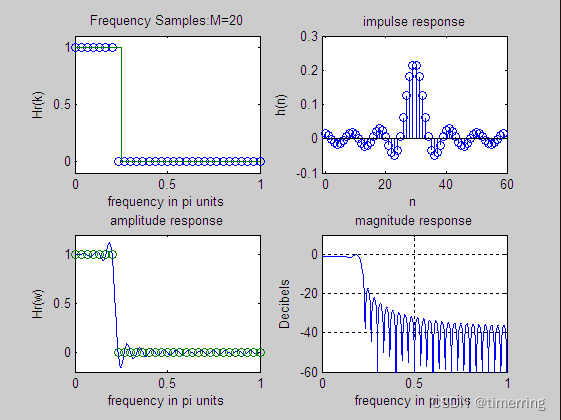展开全文matlab 频率采样法设计FIR
• 手把手教你设计FIR数字滤波器最新版-手把手教你设计FIR数字滤波器.docx 本帖最后由 leo_silverbulle 于 2015-4-22 09:38 编辑  上次发的帖忘了上传附件，楼主的锅，这是我这段时间设计FIR滤波器的过程中自己...matlab
• 【设计】FIR数字滤波器MATLAB中的实现.docx【设计】FIR数字滤波器MATLAB中的实现.docx【设计】FIR数字滤波器MATLAB中的实现.docx【设计】FIR数字滤波器MATLAB中的实现.docx【设计】FIR数字滤波器MATLAB中...
• 【设计】FIR数字滤波器MATLAB中的实现.pdf【设计】FIR数字滤波器MATLAB中的实现.pdf【设计】FIR数字滤波器MATLAB中的实现.pdf【设计】FIR数字滤波器MATLAB中的实现.pdf【设计】FIR数字滤波器MATLAB中的...
• 目录：MATLAB-FIR数字滤波器设计1 FIR滤波器的原理2 FIR滤波器的特点2.1 相位特性2.1.1 偶对称2.1.2 奇对称2.2 幅度特性3 几种滤波器函数3.1 fir1()3.2 fir2()3.3 kaiserord()3.4 firpm()4 filterDesigner配置4.1 ...matlab...